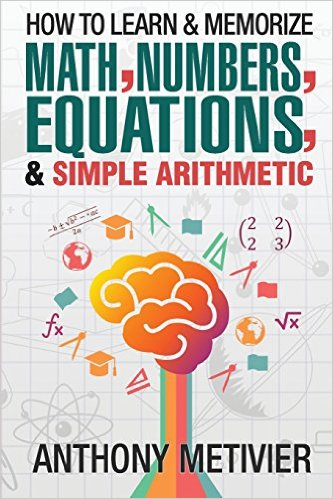# How To Learn And Memorize Math, Numbers, Equations, And Simple ArithmeticAuthor:
Genre: Study
Tags: learn math, study tips
ASIN: 1503217043

If you've ever wanted to improve your ability to learn and memorize mathematical equations, formula, arithmetic and numbers by 100% ... 200% ... 300% (or more) using simple skills you can learn in under an hour (or less), then this may be the most important book you will ever read.

You’re wasting time listening to the standard advice about learning math. You can’t continue with “random acts of learning” as you study simple math, calculus and statistics formulas – at least not for long.

The truth is that learning math and remembering numbers can be incredibly simple. You just need to know how.

In How To Memorize Numbers, Equations And Simple Arithmetic, Anthony Metivier shows you everthing you need to develop the right skills, the right mindset and the right dedicated memorization strategy for memorizing any number or equation.

The key to learning and memorizing math is to follow a model. You won’t succeed without one. And your best bet is to supplement that math learning model with strong memory skills.

Want To Eliminate The Pain and Frustration of Learning Math?

Most of the suffering caused by learning math comes from “cognitive overload.”

There is a way to remove this frustration from your life forever. And …

… If There Is A Quick Fix – This Is It!

The information in this book will teach you:

* Why memory techniques for math and numbers are like a bicycle everyone can ride (with some minor personal adjustments).

* The real reason why no one should ever be squeamish about memorization or learning math.

* Sample examples and illustrations that will show you exactly how and why these memory techniques and strategies work.

* Unique approaches that will have you literally “tuning in” on the math concepts and formulas you are studying so that you can memorize and recall them with ease.

Learn And Memorize Numbers, Equations And Formulas By The Dozens

Memorizing formulas for arithmetic, calculus, physics and statistics is one of the greatest frustrations math learners face. But using the Memory Palace and visualization secrets revealed in this book, you’ll learn:

1. How to visualize any number so that it literally pops out in your mind whenever you look for it.

2. How to use actors, other public figures and famous pieces of artwork to help you memorize math concepts, numbers and formulas.

3. Simple strategies for practicing recall so that you are relaxed during exams and can easily recall everything you’ve studied no matter how difficult.

The Best Ways To Learn And Memorize Math

What’s the secret to success with using this book to memorize all the mathematical formulas, equations and numbers that you need to excel at math? It all starts with having a “system” for doing the necessary memorization activities. And that’s why you need a proven plan for increasing your math knowledge.

The good news is it’s not hard to improve your approach to learning math.

How To Memorize Numbers, Equations And Simple Arithmetic gives you amazing tools designed to speed up the process and get you solid results. Understand how the method taught in this book works and you’ll make advanced strides in how you learn math quickly and in ways that are effective, elegant and fun.

Would You Like To Know More?